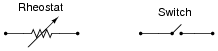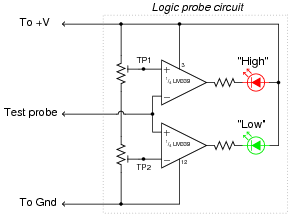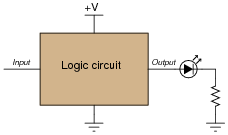# Digital Logic Signals

## Digital Circuits

• #### Question 1

A rheostat (variable resistor) and a switch are both examples of electric components exhibiting different degrees of conductivity:Which of these devices would be considered discrete and which would be considered continuous in terms of their electrical conductivity? What do each of these words mean, and how might they apply to variables in electric circuits other than conductivity?

• #### Question 2

Digital logic circuitry makes use of discrete voltage levels: each “logic gate” sub-circuit inputs and outputs voltages that are either considered “high” or “low”. Define what both of these terms means in a digital logic circuit powered by 5 volts DC.

• #### Question 3

Determine the logic levels (either “high” or “low”) at each of the test points in this circuit with the toggle switch in the open position, as well as the status of the transistor and LED:VTP1 = (high or low?)
VTP2 = (high or low?)
VTP3 = (high or low?)
VTP4 = (high or low?)
Transistor = (on or off?)
LED = (on or off?)
• #### Question 4

If we need to produce a discrete logic signal (“high” or “low”) from a mechanical switch, the most direct way of doing so is to use a single-pole, double-throw switch (SPDT) like this:In the “High” position, the switch directly connects the signal line to V, ensuring a high logic state; in the “Low” position, the switch directly connects the signal line to ground, ensuring a low logic state. What could be simpler?

However, often a SPDT switch is not feasible and we must use a SPST (single-pole, single-throw) switch instead:A problem often arises with such configurations because in the open position there is neither a connection to V nor ground. In other words, these two SPST configurations produce the exact same indeterminate logic state (“floating”) when their respective switches are open. To remedy this, resistors are often added to such circuits:Explain what functions the pulldown and pull up resistors serve, and also why they are referred to by those names.

• #### Question 5

A common mistake made by students new to digital circuits is to misplace the pull up or pulldown resistors in schematic diagrams, and also in the circuits they build. Study the following schematics and determine whether the resistor in each one is a properly-placed pull up or pulldown resistor, or if it is improperly placed:• #### Question 6

A logic probe is a very useful tool for working with digital logic circuits. It indicates “high” and “low” logic states by means of LED’s, giving visual indication only if the voltage levels are appropriate for each state.

Here is a schematic diagram for a logic probe built using comparators. Each comparator has a threshold adjustment potentiometer, so that it may be set to indicate its respective logic state only if the signal voltage is well within the range stated by the logic manufacturer:Explain how this circuit functions.

• #### Question 7

Identify whether each of these quantities is continuous or discrete:

Resistance of a rheostat:
Resistance of a switch:
Time represented by an analog clock:
Time represented by a digital clock:
Quantity of money in a billfold (bills and coins):
Number of pebbles held in a hand:
A person’s weight, in pounds or kilograms:
Voltage output by a comparator:
Voltage output by an operational amplifier:
Electrical conductivity of a thyristor:
• #### Question 8

Two computational aids of antiquity are the abacus and the slide rule. Which of these mathematical instruments would be considered “analog” and which would be considered “digital”? Explain your answer.

• #### Question 9

In digital electronic circuitry, binary bit values of 0 or 1 are represented in the form of voltages: low and high logic states, respectively. Suppose you need to manually “input” a logic state to one of the pins of a logic circuit. In the following illustration, the logic circuit (shown as an indistinct, shaded rectangle) is already supplied with DC power ( V and ground), and its output is indicated by an LED. All it requires is an input from you:Complete this schematic diagram by including a switch in the drawing, such that in each of its two positions, a definite “low” or “high” logic state will be sensed by the circuit’s input terminal.